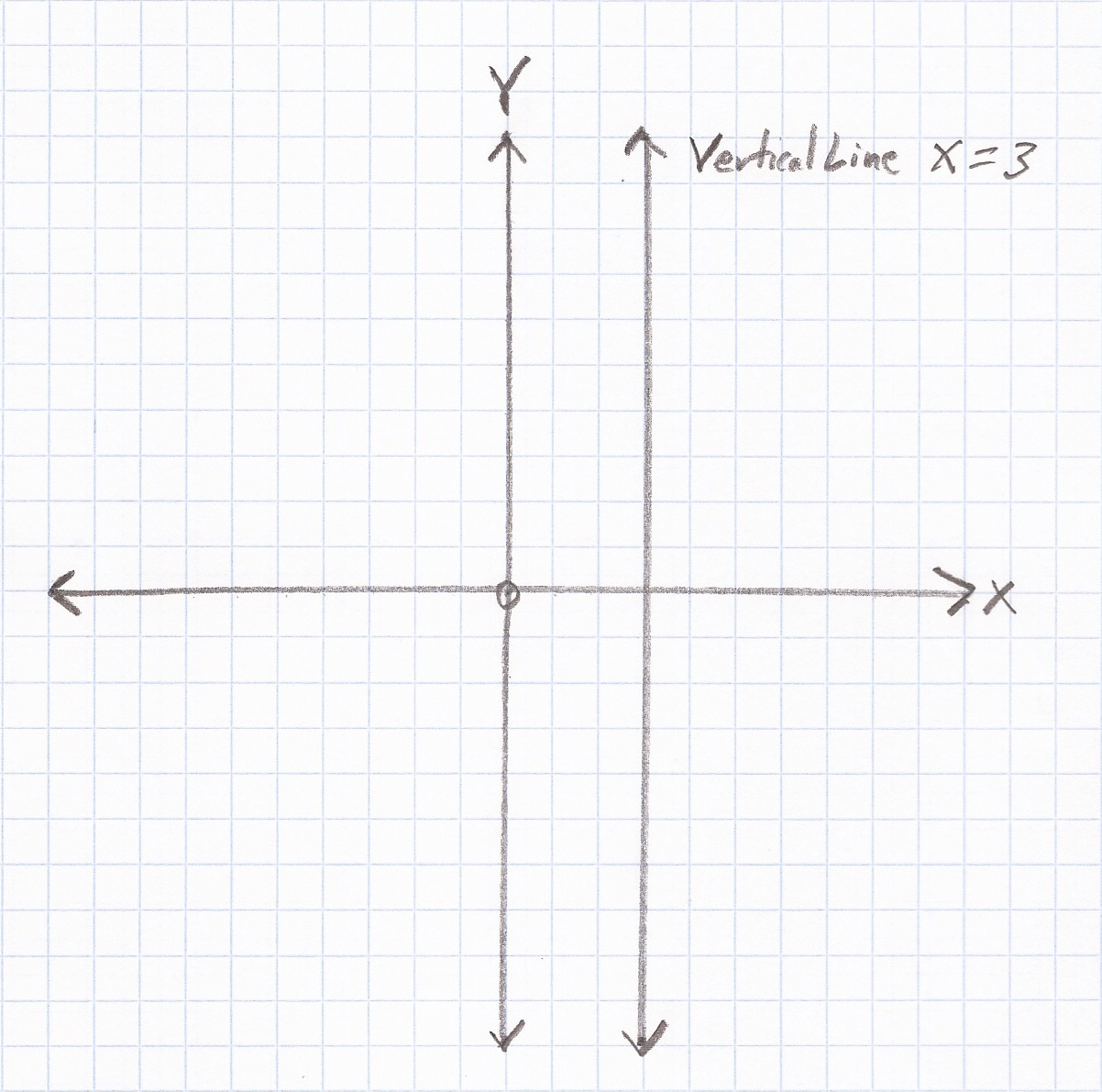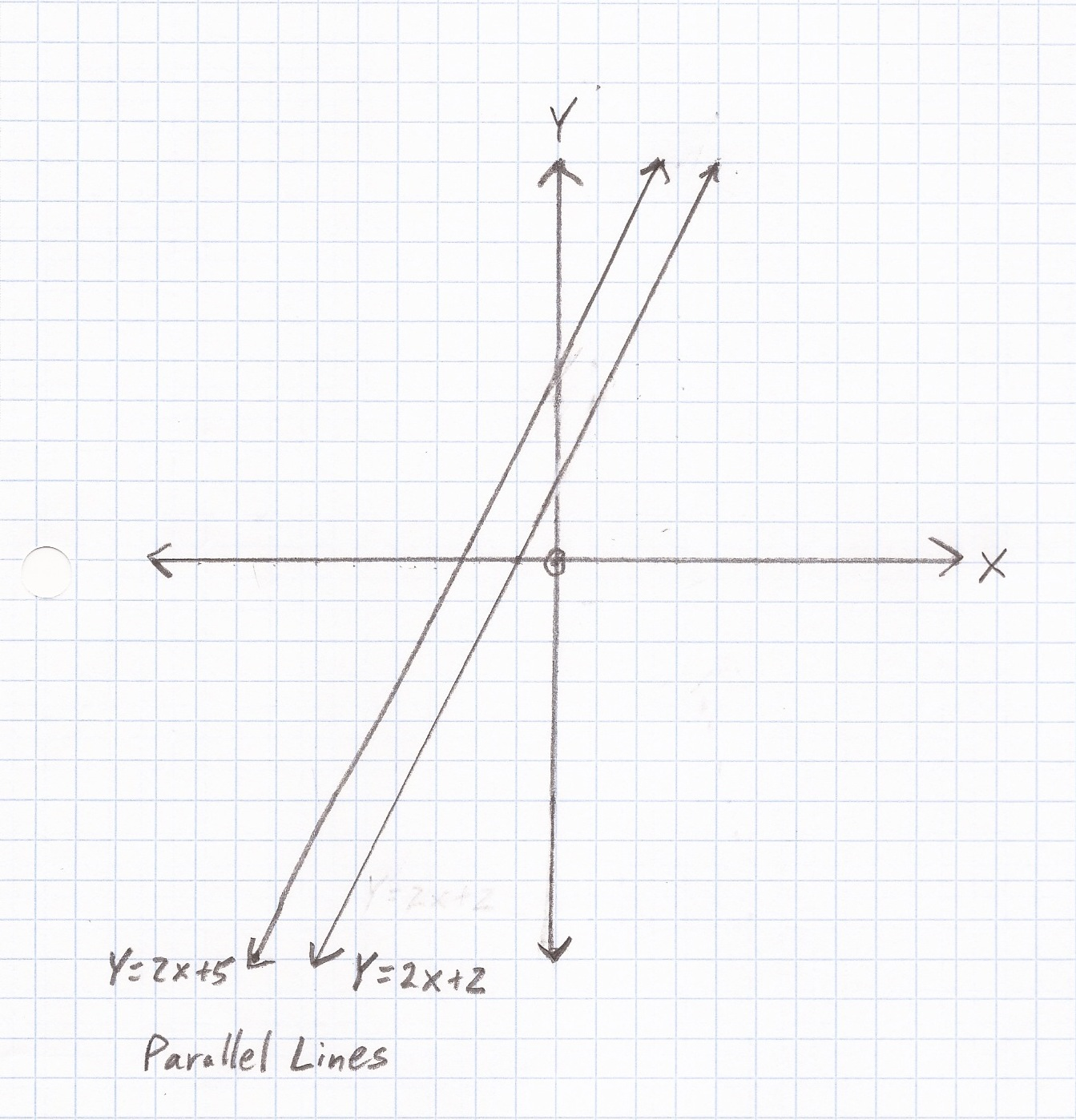## Slope Specials

Vertical, Horizontal, Parallel, Perpendicular

Slope of a Vertical Line
Undefined
x = 3Slope of a Horizontal Line

Zero
y = 3Slope of Parallel Lines

Equal (parallel lines never cross each other)
Because m = 2 for both lines,  y = 2x + 2 is parallel to y = 2x + 5Slope of Perpendicular Lines

Negative Inverse (perpendicular lines cross at a right angle to each other)
Because 2 and –$\bf\displaystyle\frac{1}{2}$ are negative inverses, y = 2x + 2 is perpendicular to y = –$\bf\displaystyle\frac{1}{2}$ x + 4Question

What is the slope of x = 7

Undefined

Question

What is the slope of y = 8

0

Question

The equations of two lines are y = -9x +4 and y = -9x +7, respectively.
True or False: Are these lines parallel?

True

Because the slopes of the two lines are the same, -9, the lines are parallel.

Question

The equations of two lines are y = –$\bf\displaystyle\frac{1}{8}$ x + 4 and y = 8x – 3, respectively.
True or False: Are these lines perpendicular?

True

Because the slopes of the two lines are negative inverses, –$\bf\displaystyle\frac{1}{8}$ and 8, the lines are perpendicular.

Practice – Questions

1.  What is the slope of x = -7?

2.  What is the slope of y = -3?

3.  The equations of two lines are y = 7x + 5 and y = 7x – 3, respectively.
True or False: Are these lines parallel?

4.  The equations of two lines are y = –$\bf\displaystyle\frac{1}{3}$ x + 9 and y = 3x – 6, respectively.
True or False: Are these lines perpendicular?

5.  The equations of two lines are y =$\bf\displaystyle\frac{1}{5}$ x + 2 and y = -5x – 4, respectively.
True or False: Are these lines perpendicular?